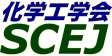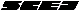## \$B9V1i%W%m%0%i%`!J2q>l!&F|DxJL!K(B

### XC\$B2q>l(B \$BBh(B2\$BF|(B

\$B:G=*99?7F|;~!'(B2012-08-20 15:02:17
\$B9V1i(B
\$B;~9o(B
\$B9V1i(B
\$BHV9f(B
\$B9V1iBjL\!?H/I=\$B%-!<%o!<%I(B\$BJ,N`(B
\$BHV9f(B
\$B\$BHV9f(B
\$B%7%s%]%8%&%`(B \$B!c
9:00\$B!A(B 12:00XC2P01\$B%R!<%H%]%s%W<01xE%4%Ag5!(B
(\$B?@9]4D6-%=%j%e!<%7%g%s(B) \$B!{:4F#(B \$BJ~90(B
heat pump
sludge treatment
dehumidification drier
S-24145
9:00\$B!A(B 12:00XC2P02\$B9bF)2a@-%<%*%i%\$%HKl%(%l%a%s%H\$N
(\$BF|N)B\$A%(B) \$B!{ \$B!&(B \$BLpLn(B \$BOB9((B \$B!&(B \$B_7B<(B \$B7r0l(B \$B!&(B \$BF#ED(B \$BM%(B \$B!&(B \$B9bLZ(B \$B5A?.(B \$B!&(B \$B@uMx(B \$B>M9-(B \$B!&(B \$B9bFi(B \$B9@Fs(B \$B!&(B \$BLpLn(B \$B=_(B \$B!&(B \$BAj_7(B \$B@5?.(B
separation
membrane
zeolite
S-24146
9:00\$B!A(B 12:00XC2P03Innovative design concept for spiral wound element \$B!H(BLD TechnologyTM\$B!I(B
(\$BF|ElEE9)(B) \$B!{LSMx(B \$BD> \$B!&(B \$B:g86(B \$B9/G7(B \$B!&(B \$B@nEg(B \$BIR9T(B \$B!&(B \$B?7C+(B \$BBn;J(B \$B!&(B \$BW"@%(B \$B2mI'(B
bio-fouling
lower differential pressure
energy save
S-24160
9:00\$B!A(B 12:00XC2P04\$B%?%s%8%'%s%7%c%k%U%m!<%U%#%k%H%l!<%7%g%s\$K\$*\$1\$k\$m2a>r7o\$HAK;_N((B
(\$B%a%k%/(B) \$B!{@n>e(B \$BBY9-(B \$B!&(B (\$B@5(B)\$B5WJ]ED(B \$BCN;R(B \$B!&(B (\$B@5(B)\$B0KF#(B \$BN4IW(B
cross flow
membrane separation
rejection
S-24180
9:00\$B!A(B 12:00XC2P05\$B%^%\$%/%m%P%V%k\$rMQ\$\$\$?Kl\$m2a>t?e=hM}5;=Q(B
(\$B%@%\$%;%s!&%a%s%V%l%s!&%7%9%F%`%:(B) \$B!{(B(\$B6&(B)\$BLJIt(B \$BCR0l(B \$B!&(B (\$B6&(B)\$BCfDM(B \$B=\$;V(B
microbubble
membranefiltration
celluloseacetate
S-24190
9:00\$B!A(B 12:00XC2P06\$BGS?eMQCf6u;e7?Dc05(BRO\$BKl%b%8%e!<%k(B
(\$BElMNKB(B) \$B!{NS(B \$BLP \$B!&(B \$B0KF#(B \$BM5Fs(B \$B!&(B (\$B@5(B)\$B7'Ln(B \$B=_IW(B \$B!&(B (\$B@5(B)\$BH,LZ(B \$BIR9,(B
Hollow fiber RO
Cellulose triacetate
Superior fouling resistance
S-24254
9:00\$B!A(B 12:00XC2P07\$BC1AX<+N)7?Bg8}7B(BUF\$BKl\$N3+H/\$H9bByEYGS?e\$NFb05=hM}FC@-(B
(\$B@Q?e2=3X(B) \$B!{6L0f(B \$B=SMN(B \$B!&(B \$BJ!0f(B \$BM\$(B \$B!&(B \$BC+B<(B \$B:i(B \$B!&(B \$B>>Hx(B \$BN60l(B \$B!&(B \$BBg?y(B \$B9b;V(B
membrane
waste water treatment
in-to-out operation
S-24322
9:00\$B!A(B 12:00XC2P08\$BFbItG.8r497?%P%C%A>xN1\$N
(\$B;04]2=3X(B) \$B!{(B(\$B3X(B)\$BNkLZ(B \$BBYI'(B \$B!&(B (\$B@5(B)\$B:{^<(B \$BM[(B \$B!&(B (\$B;37ABg1!M}9)(B) (\$B3X(B)\$B;3LZ(B \$BM:Bg(B \$B!&(B (\$B@5(B)\$B>>ED(B \$B7=8g(B
Batch distillation
Heat-integration
Energy saving
S-24702
9:00\$B!A(B 12:00XC2P09\$B?;DR7?J?Kl(BMBR\$B\$N;:6HGS?e=hM}E,MQ(B
(\$B%/%\%?(B) \$B!{FAEg(B \$B44<#(B \$B!&(B \$BBg0f(B \$BM5N<(B \$B!&(B \$B4dAR(B \$B0l@8(B
MBR
Membrane
S-24740

\$B9V1i%W%m%0%i%`(B
\$B2=3X9)3X2q(B \$BBh(B44\$B2s=)5(Bg2q(B(C) 2012 \$B8x1W
Most recent update: 2012-08-20 15:02:17
For more information contact \$B2=3X9)3X2qElKL;YIt(B \$BBh(B44\$B2s=)5(Bg2q(B \$BLd\$\$9g\$;78(B
E-mail: inquiry-44fwww3.scej.org
This page was generated byeasp 2.28; proghtml 2.28c (C)1999-2011 kawase